# 290 N The Unknown Component Problem Lecture 10

• Slides: 8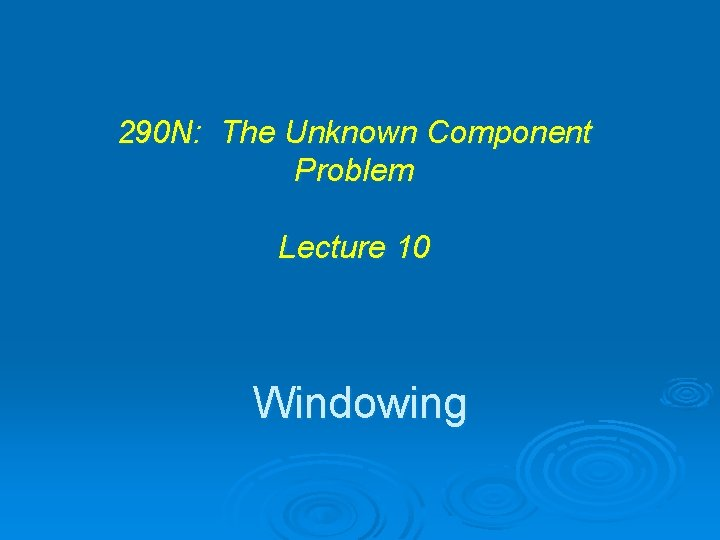290 N: The Unknown Component Problem Lecture 10 Windowing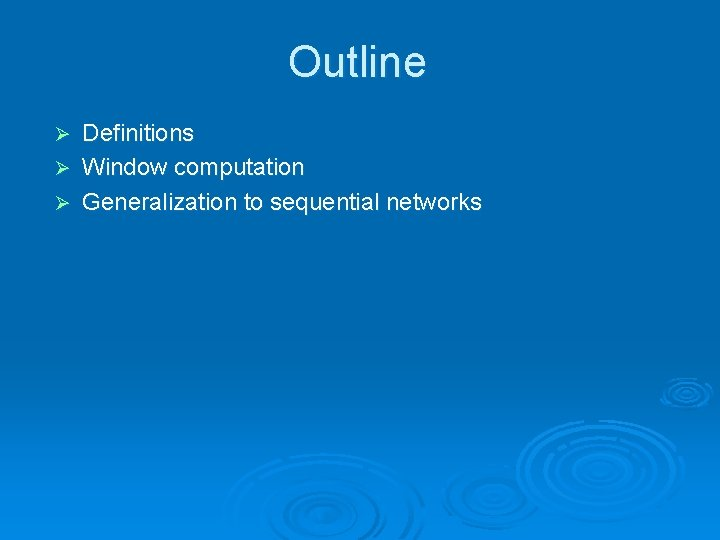Outline Definitions Ø Window computation Ø Generalization to sequential networks Ø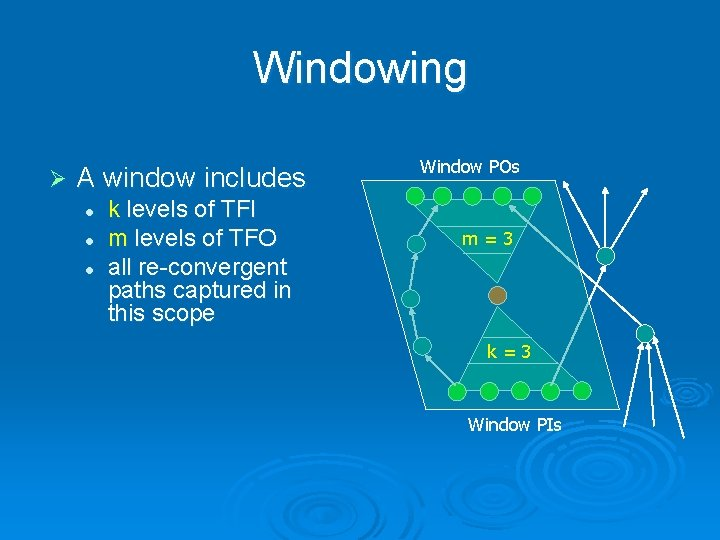Windowing Ø A window includes l l l k levels of TFI m levels of TFO all re-convergent paths captured in this scope Window POs m=3 k=3 Window PIsDefinition of Leaves and Roots Ø Definition. Given a directed acyclic graph and two non-overlapping subsets of its nodes, one set is called the leaf set and the other the root set, if every path from any node in the root set towards the sources of the graph passes through some node in the leaf set.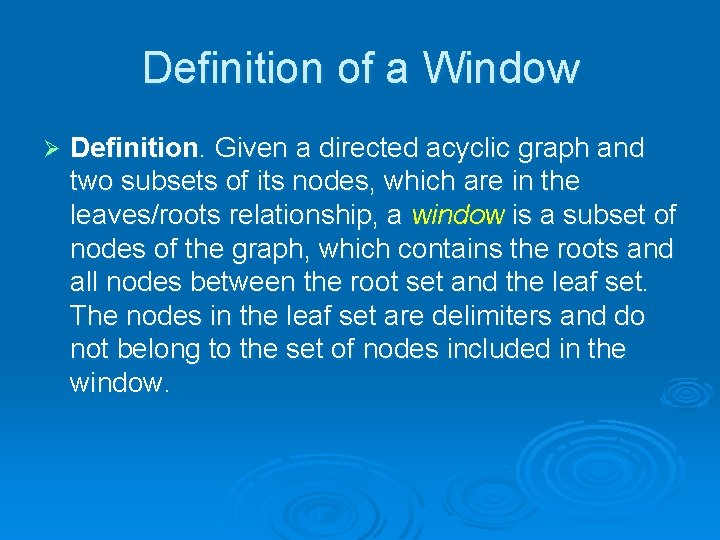Definition of a Window Ø Definition. Given a directed acyclic graph and two subsets of its nodes, which are in the leaves/roots relationship, a window is a subset of nodes of the graph, which contains the roots and all nodes between the root set and the leaf set. The nodes in the leaf set are delimiters and do not belong to the set of nodes included in the window.Definition of Distance Definition. A path between a pair of nodes is distance-k if it spans exactly k edges between the pair. Ø Definition. Two nodes are distance-k from each other if Ø l l there exists a distance-k path between them, and all other paths between them are distance-k or less.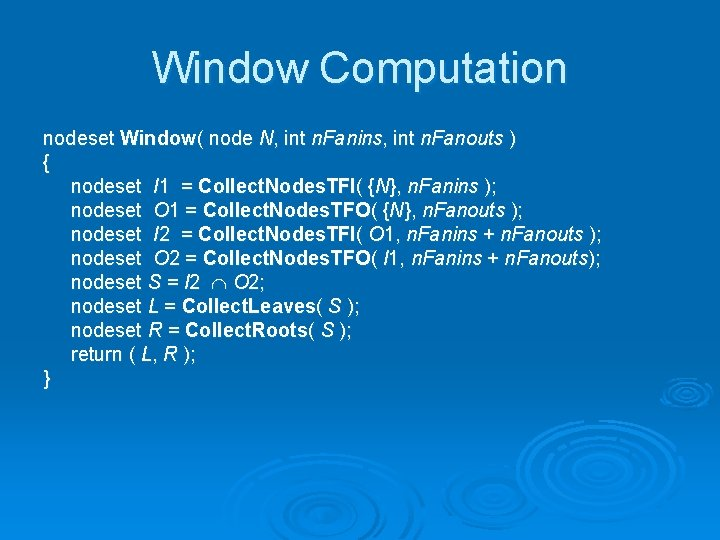Window Computation nodeset Window( node N, int n. Fanins, int n. Fanouts ) { nodeset I 1 = Collect. Nodes. TFI( {N}, n. Fanins ); nodeset O 1 = Collect. Nodes. TFO( {N}, n. Fanouts ); nodeset I 2 = Collect. Nodes. TFI( O 1, n. Fanins + n. Fanouts ); nodeset O 2 = Collect. Nodes. TFO( I 1, n. Fanins + n. Fanouts); nodeset S = I 2 O 2; nodeset L = Collect. Leaves( S ); nodeset R = Collect. Roots( S ); return ( L, R ); }Example O 1 S S O 1 N S S I 1